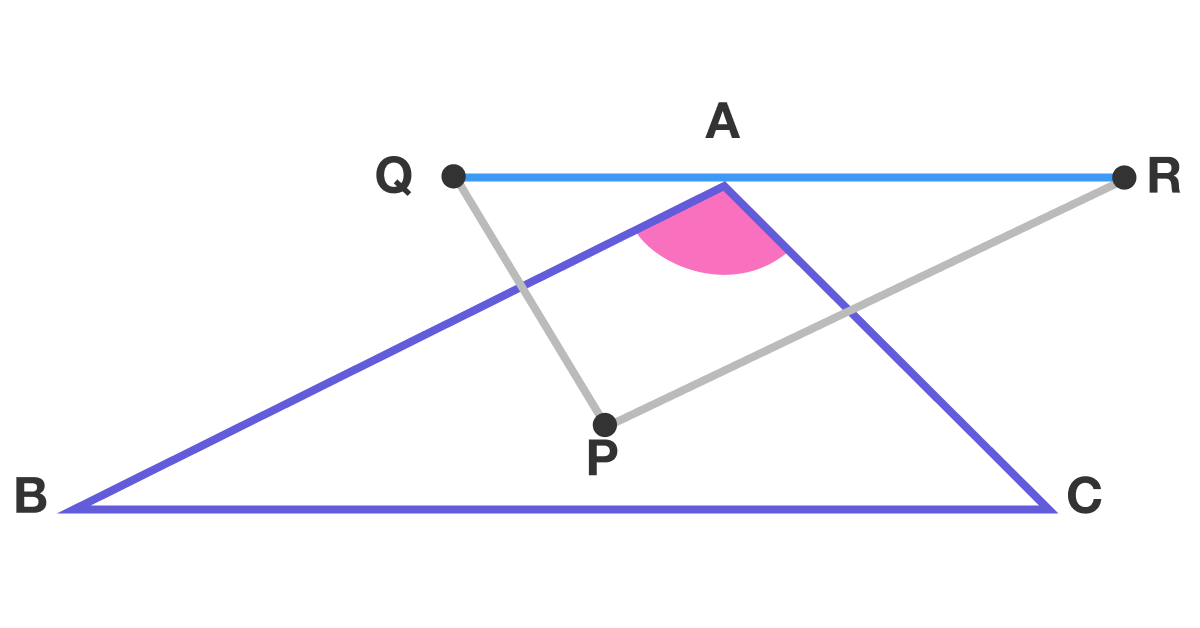# Why this type of triangle has more properties?

Geometry Level 1

Let $P$ be an interior point of triangle $ABC$.
Let $Q$ and $R$ be the reflections of $P$ in $AB$ and $AC$, respectively.

If $QAR$ is a straight line, find $\angle BAC$ in degrees.The image is not drawn to scale.

×